# Linear Impulse Momentum Relations (Part - 2) Civil Engineering (CE) Notes | EduRev

## Civil Engineering (CE) : Linear Impulse Momentum Relations (Part - 2) Civil Engineering (CE) Notes | EduRev

The document Linear Impulse Momentum Relations (Part - 2) Civil Engineering (CE) Notes | EduRev is a part of the Civil Engineering (CE) Course Introduction to Dynamics and Vibrations- Notes, Videos, MCQs.
All you need of Civil Engineering (CE) at this link: Civil Engineering (CE)

Example 4 Rocket propulsion equations. Rocket motors and jet engines exploit the momentum conservation law in order to produce motion. They work by expelling mass from a vehicle at very high velocity, in a direction opposite to the motion of the vehicle. The momentum of the expelled mass must be balanced by an equal and opposite change in the momentum of the vehicle; so the velocity of the vehicle increases.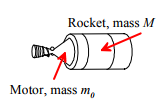Analyzing a rocket engine is quite complicated, because the propellant carried by the engine is usually a very significant fraction of the total mass of the vehicle. Consequently, it is important to account for the fact that the mass decreases as the propellant is used.

Assumptions:

1. The figure shows a rocket motor attached to a rocket with mass M.

2. The rocket motor contains an initial mass m0 of propellant and expels propellant at rate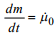with a velocity v0 = -v0i  relative to the rocket.
3. We assume straight line motion, and assume that no external forces act on the rocket or motor.

Calculations:

The figure shows the rocket at an instant of time t, and then a very short time interval dt later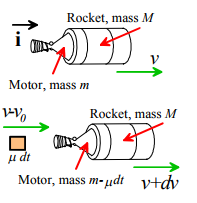1. At time t, the rocket moves at speed v, and the system has momentum P = (M + m)vi where m is the motor’s mass.
2. During the time interval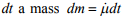is expelled from the motor. The velocity of the expelled mass is v = (v-v0)i.
3. At time t+dt the mass of the motor has decreased to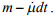4. At time t+dt, the rocket has velocity v = (v + dv)i.
5. The total momentum of the system at time t+dt is therefore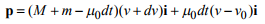6. Momentum must be conserved, so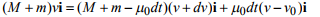7. Multiplying this out and simplifying shows that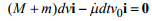where the term μ0dtdv has been neglected.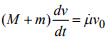This result is called the `rocket equation.’

Specific Impulse of a rocket motor: The performance of a rocket engine is usually specified by its `specific impulse.’ Confusingly, two different definitions of specific impulse are commonly used: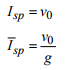The first definition quantifies the impulse exerted by the motor per unit mass of propellant; the second is the impulse per unit weight of propellant. You can usually tell which definition is being used from the units – the first definition has units of m/s; the second has units of s. In terms of the specific impulse, the rocket equation is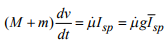Integrated form of the rocket equation: If the motor expels propellant at constant rate, the equation of motion can be integrated. Assume that

1. The rocket is at the origin at time t=0;
2. The rocket has speed v0 at time t=0

3. The motor has mass m0 at time t=0; this means that at time t it has mass m0 - μt Then the rocket’s speed can be calculated as a function of time: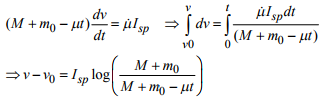Similarly, the position follows as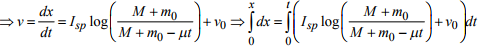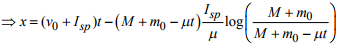These calculations assume that no external forces act on the rocket. It is quite straightforward to generalize them to account for external forces as well – the details are left as an exercise.

Example 5 Application of rocket propulsion equation: Calculate the maximum payload that can be launched to escape velocity on the Ares I launch vehicle.

‘Escape velocity’ means that after the motor burns out, the space vehicle can escape the earth’s gravitational field – see example 5 in Section 4.1.6.

Assumptions

1. The specifications for the Ares I are at Relevant variables are listed in the table below.

 Total mass (kg) Specific impulse (s) Propellant mass (kg) Stage I 586344 268.8 5o4516 Stage II 183952 452.1 163530

2. As an approximation, we will neglect the motion of the rocket during the burn, and will neglect aerodynamic forces.
3. We will assume that the first stage is jettisoned before burning the second stage.
4. Note that the change in velocity due to burning a stage can be expressed as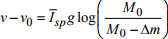where M0 is the total mass before the burn, and Δm is the mass of propellant burned.

5. The earth’s radius is 6378.145km
6. The Gravitational parameter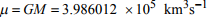(G= gravitational constant; M=mass of earth)
7. Escape velocity is from the earths surface is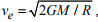where R is the earth’s radius.

Calculation:

1. 1. Let m denote the payload mass; let m1, m2 denote the total masses of stages I and II, let m01, m02 denote the propellant masses of stages I and II; and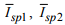denote the specific impulses of the two stages.
2. The rocket is at rest before burning the first stage; and its total mass is m + m1 + m2 + m01 + m02 . After burn, the mass is m + m1 + m2 + m02 . The velocity after burning the first stage is therefore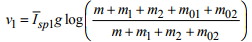3. The first stage is then jettisoned – the mass before starting the second burn is m + m2 + m02 , , and after the second burn it is m + m2 . The velocity after the second burn is therefore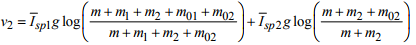4. Substituting numbers into the escape velocity formula gives ve =  km/sec. Substituting numbers for the masses shows that to reach this velocity, the payload mass must satisfy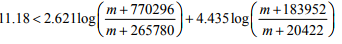where m is in kg.

5. We can use MAPLE to solve for the critical value of m for equality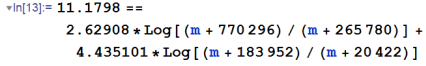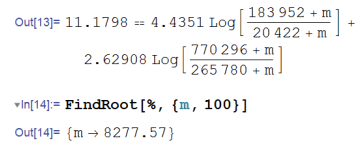so the solution is 8300kg – a very small mass compared with that of the launch vehicle, but you could pack in a large number of people you would like to launch into outer space nonetheless (the entire faculty of the division of engineering, if you wish).

4.2.7 Analyzing collisions between particles: the restitution coefficient

The momentum conservation equations are particularly helpful if you want to analyze collisions between two or more objects. If the impact occurs over a very short time, the impulse exerted by the contact forces acting at the point of collision is huge compared with the impulse exerted by any other forces. If we consider the two colliding particles as a system, the external impulse exerted on the system can be taken to be zero, and so the total momentum of the system is conserved.

The momentum conservation equation can be used to relate the velocities of the particles before collision to those after collision. These relations are not enough to be able to determine the velocities completely, however – to do this, we also need to be able to quantify the energy lost (or more accurately, dissipated as heat) during the collision.

In practice we don’t directly specify the energy loss during a collision – instead, the relative velocities are related by a property of the impact called the coefficient of restitution.

Restitution coefficient for straight line motion

Suppose that two colliding particles A and B move in a straight line parallel to a unit vector i. Let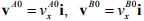denote the velocities of A and B just before the collision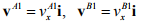denote the velocities of A and B just after the collision.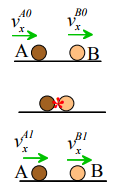The velocities before and after impact are related by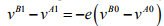where e is the restitution coefficient. The negative sign is needed because the particles approach one another before impact, and separate afterwards.

Restitution coefficient for 3D frictionless collisions

For a more general contact, we define

VA0 VB0 to denote velocities of the particles before collision

VA1 VB1 to denote velocities of the particles after collision

In addition, we let n be a unit vector normal to the common tangent plane at the point of contact (if the two colliding particles are spheres or disks the vector is parallel to the line joining their centers).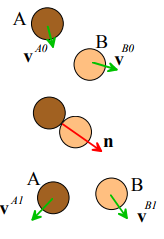The velocities before and after impact are related by two vector equations: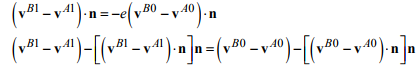To interpret these equations, note that
1. The first equation states that the component of relative velocity normal to the contact plane is reduced by a factor e (just as for 1D contacts)
2. The second equation states that the component of relative velocity tangent to the contact plane is unchanged (this is because the contact is frictionless, and so the reaction forces acting on the particles during the collision exert no impulse is exerted in a direction tangent to the contact plane).

The two equations can be combined into a single vector equation relating velocities before and after impact – this form is more compact, and often more useful, but more difficult to visualize physically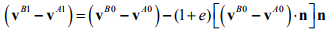It is possible to account for friction at the contact, but friction will always make the colliding particles rotate, so they cannot be idealized as particles.

Values of restitution coefficient

The restitution coefficient almost always lies in the range 0 < e < 1 . It can only be less than zero if one object can penetrate and pass through the other (e.g. a bullet); and can only be greater than 1 if the collision generates energy somehow (e.g. releasing a preloaded spring, or causing an explosion).

If e=0, the two colliding objects stick together; if e=1 the collision is perfectly elastic, with no energy loss.

The restitution coefficient is strongly sensitive to the material properties of the two colliding objects, and also varies weakly with their geometry and the velocity of impact. The two latter effects are usually ignored.

Collision between a particle and a fixed rigid surface. The collision formulas can be applied to impact between a rigid fixed surface by taking the surface to be particle B, and noting that the velocity of particle B is then zero both before and after impact.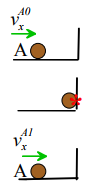For straight line motion  vA1 = -evA0

For collision with an angled wall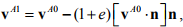where n is a unit vector perpendicular to the wall.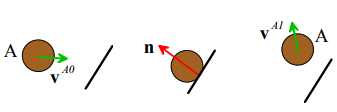4.2.8 Examples of collision problems

Example 1 Suppose that a moving car hits a stationary (parked) vehicle from behind. Derive a formula that will enable an accident investigator to determine the velocity of the moving car from the length of the skid marks left on the road.

Assumptions:

1. We will assume both cars move in a straight line
2. The moving and stationary cars will be assumed to have masses  m1, m2 , respectively
3. We will assume the cars stick together after the collision (i.e. the restitution coefficient is zero)
4. We will assume that only the parked car has brakes applied after the collision

This calculation takes two steps: first, we will use work-energy to relate the distance slid by the cars after impact to their velocity just after the impact occurs. Then, we will use momentum and the restitution formula to work out the velocity of the moving car just before impact.

Calculation: Let V denote the velocity of the moving car just before impact; let v1 denote the velocity of the two (connected) cars just after impact, and let d denote the distance slid.

1. The figure shows a free body diagram for each of the two cars during sliding after the collision. Newton’s law of motion for each car shows that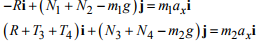2. The vertical component of the equations of motion give N1 + N2 = m1g ; N3 + N4 - m2g .

3. The parked car has locked wheels and skids over the road; the friction law gives the tangential forces at the contacts as T3 + T4 = μ (N3 + N4) = μm2g

4. We can calculate the velocity of the cars just after impact using the work-kinetic energy relation during skidding. For this purpose, we consider the two connected cars as a single particle. The work done on the particle by the friction forces is - (T3 + T4) d = -μm2gd . The work done is equal to the change in kinetic energy of the particle, so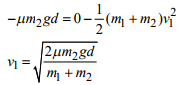5. Finally, we can use momentum conservation to calculate the velocity just before impact. The momentum after impact is h1 = (m1 +m2)v1i, while before impact h0 = m1Vi. Equating the two gives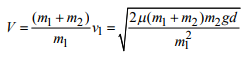Offer running on EduRev: Apply code STAYHOME200 to get INR 200 off on our premium plan EduRev Infinity!

## Introduction to Dynamics and Vibrations- Notes, Videos, MCQs

20 videos|53 docs

,

,

,

,

,

,

,

,

,

,

,

,

,

,

,

,

,

,

,

,

,

;